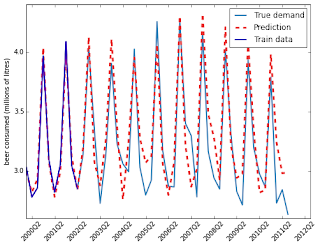Over a million developers have joined DZone.{{announcement.body}}
{{announcement.title}}

DZone's Guide to

# Forecasting Beer Consumption with Sklearn

· Big Data Zone ·
Free Resource

Comment (0)

Save
{{ articles.views | formatCount}} Views

How to Simplify Apache Kafka. Get eBook.

In this post we will see how to implement a straightforward forecasting model based on the linear regression object of sklearn. The model that we are going to build is based on the idea idea that past observations are good predictors of a future value. Using some symbols, given xn−k,...,xn−2,xn−1 we want to estimate xn+h where h is the forecast horizon just using the given values. The estimation that we are going to apply is the following:where xn−k and xn−1 are respectively the oldest and the newest observation we consider for the forecast. The weights wk,...,w1,w0 are chosen in order to minimizewhere m is number of periods available to train our model. This model is often referred as regression model with lagged explanatory variables and k is called lag order.

Before implementing the model let's load a time series to forecast:

```import pandas as pd
to_forecast = df.TotalBeer.values
dates = df.DATE.values```

The time series represent the total of alcohol consumed by quarter millions of litres from the 1st quarter of 2000 to 3rd quarter of 2012. The data is from New Zealand government and can be downloaded in csv from here. We will focus on the forecast of beer consumption.

First, we need to organize our data in forecast in windows that contain the previous observations:

```import numpy as np

def organize_data(to_forecast, window, horizon):"""
Input:
to_forecast, univariate time series organized as numpy array
window, number of items to use in the forecast window
horizon, horizon of the forecast
Output:
X, a matrix where each row contains a forecast window
y, the target values for each row of X
"""
shape = to_forecast.shape[:-1]+/(to_forecast.shape[-1]- window +1, window)
strides = to_forecast.strides +(to_forecast.strides[-1],)
X = np.lib.stride_tricks.as_strided(to_forecast,
shape=shape,
strides=strides)
y = np.array([X[i+horizon][-1]for i in range(len(X)-horizon)])return X[:-horizon], y

k =4# number of previous observations to use
h =1# forecast horizon
X,y = organize_data(to_forecast, k, h)```

Now, X is a matrix where the i-th row contains the lagged variables xn−k,...,xn−2,xn−1 and y[i] contains the i-th target value. We are ready to train our forecasting model:

```from sklearn.linear_model importLinearRegression

m =10# number of samples to take in account
regressor =LinearRegression(normalize=True)
regressor.fit(X[:m], y[:m])```

We trained our model using the first 10 observations, which means that we used the data from 1st quarter of 2000 to the 2nd quarter of 2002. We use a lag order of one year and a forecast horizon of 1 quarter. To estimate the error of the model we will use the mean absolute percentage error (MAPE). Computing this metric to compare the forecast of the remaining observation of the time series and the actual observations we have:

```def mape(ypred, ytrue):""" returns the mean absolute percentage error """
idx = ytrue !=0.0return100*np.mean(np.abs(ypred[idx]-ytrue[idx])/ytrue[idx])print'The error is %0.2f%%'% mape(regressor.predict(X[m:]),y[m:])```
```The error is 6.15%
```

Which means that, on average, the forecast provided by our model differs from the target value only of 6.15%. Let's compare the forecast and the observed values visually:

```figure(figsize=(8,6))
plot(y, label='True demand', color='#377EB8', linewidth=2)
plot(regressor.predict(X),'--', color='#EB3737', linewidth=3, label='Prediction')
plot(y[:m], label='Train data', color='#3700B8', linewidth=2)
xticks(arange(len(dates))[1::4],dates[1::4], rotation=45)
legend(loc='upper right')
ylabel('beer consumed (millions of litres)')
show()```We note that the forecast is very close to the target values and that the model was able to learn the trends and anticipate them in many cases.

12 Best Practices for Modern Data Ingestion. Download White Paper.

Topics:

Comment (0)

Save
{{ articles.views | formatCount}} Views

Published at DZone with permission of

Opinions expressed by DZone contributors are their own.

# {{ parent.title || parent.header.title}}

{{ parent.tldr }}

{{ parent.urlSource.name }}